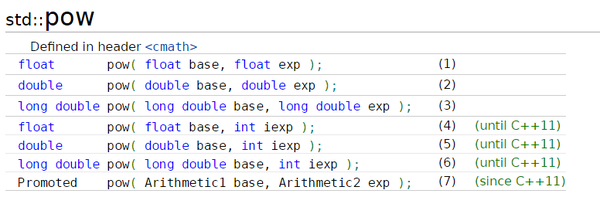• C++pow函数
2021-04-17 09:14:07
• pow(a, b): a的b次方
更多相关内容
• ## c++中pow函数

万次阅读 2016-11-15 10:38:20
C++中pow有多个重载函数； 在devpow（int，int）可以执行，但是在别的地方是不可以被编译的；会提示 ：error C2668: “pow”: 对重载函数的调用不明确 可以看见，是没有pow（int，int）这个方法的...

在C++中，pow有多个重载函数；

在dev中，pow（int，int）可以执行，但是在别的地方是不可以被编译的；会提示 ：error C2668: “pow”: 对重载函数的调用不明确可以看见，是没有pow（int，int）这个方法的；

正确的办法是pow（int，double（int））；

展开全文• 在Visio Studio 2019查找的pow函数原型为：有float和long double两种类型；以及可能出现的问题：数据类型使用不当

在Visio Studio 2019中查找的pow函数原型为：有float和long double两种类型。

float pow(_In_ float _Xx, _In_ float _Yx)；

long double pow(_In_ long double _Xx, _In_ long double _Yx)；

可能会出现的问题：

数据类型使用不当：

举例：当使用不同类型变量接收pow函数的值。

#include<iostream>
#include<math.h>
#include<typeinfo>

using namespace std;

int main()
{
int a;
long int b;
long long int c;
a = pow(2, 60);
b = pow(2, 60);
c = pow(2, 60);

cout << pow(2, 60) << endl;
cout << a << endl;
cout << b << endl;
cout << c << endl;
cout << typeid(c).name() << endl;

return 0;
}

输出为：

1.15292e+18
a=-2147483648
b=-2147483648
c=1152921504606846976
a_type=int
b_type=long
c_type=__int64
展开全文• ## C++ 【pow函数】

千次阅读 2018-08-24 11:03:06
C++中pow有多个重载函数； 在devpow（int，int）可以执行，但是在别的地方是不可以被编译的；会提示 ：error C2668: “pow”: 对重载函数的调用不明确 可以看见，是没有pow（int，int）这个方法的； ...

在C++中，pow有多个重载函数；

在dev中，pow（int，int）可以执行，但是在别的地方是不可以被编译的；会提示 ：error C2668: “pow”: 对重载函数的调用不明确可以看见，是没有pow（int，int）这个方法的；

正确的办法是pow（int，double（int））；

展开全文• ## c++pow函数用法

千次阅读 2019-09-29 00:24:46
对用户输入的任一整数，输出以下多项式...重点是掌握pow函数用法 pow(m，n)； m表示整型变量名，n表示次幂。 #include<iostream> #include<math.h> int main() { int a; std::cin>>a; std::cou...
• C++中pow()函数返回值问题 在输出pow（）计算结果时，出现越界的情况。 但先用一个long变量接收pow（）的结果，则不会出现越界。 下面展示一下我在进行梅森素数练习的时候出现的问题。 if(flag == 0) { count++; m =...
• C++ pow() 函数使用方法及示例pow()函数计算幂。此函数在头文件定义。[数学]baseexponent=pow(base,exponent)[C++语言]pow()原型[从C ++ 11标准开始]doublepow(doublebase,doubleexponent);floatpow(floatbase,...
• ## c++中的pow（）函数

万次阅读 2019-05-12 17:13:04
定义： #include <math.h> double pow( double base, double ...The pow() function returns base raised to the expth power. There’s a domain error if base is zero and exp is less than or equal to ze...
• 踩了个雷，随手以记之。 在没有超出数据范围的时候，pow计算某些质数还是会出现问题。 但是不报错。比如131。 代码和结果如图：
• ## C++中pow()函数

万次阅读 多人点赞 2019-07-21 13:42:23
函数名称:pow() 函数原型:double pow( double x, double y ); 函数功能:计算x的y次幂 所属文件:<math.h> pow(x , y) ,x的y次幂 说明：本文为个人学习笔记所用，参考网上以及书籍资料整理，不定期修改...
• c+pow函数的头文件 C ++ pow()函数 (C++ pow() function) pow() function is a library function of cmath header (<math.h> in earlier versions), it is used to find the raise to the power, it accepts ...python java javascript
• pow()函数的原型为： #include &lt;math.h&gt; double pow( double base, double exp ); The pow() function returns base raised to the expth power. There's a domain error if base is ...
• C++中pow有多个重载函数； 在devpow（int，int）可以执行，但是在别的地方是不可以被编译的；会提示 ：error C2668: “pow”: 对重载函数的调用不明确 可以看见，是没有pow（int，int）这个方法的； ...
• ## C++中关于pow函数的坑点

千次阅读 多人点赞 2019-05-14 23:30:32
pow函数C++里很好用的一个工具，可用于求一个数x的y次幂(次方) #include<cmath> //必要头文件 pow(x,y); //x的y次方 但初学者一定要慎用pow函数，因为它其实很坑… 最常见的误用应该就是pow(int,int)了，在...
• 函数原型:double pow( double x, double y ); 函数功能:计算x的y次幂 所属文件:<math.h> 如pow(2,2)意为2的2次方即为4 也可接受三个参数，如pow(2,4,5),意为2的4次方再对5取余，故输出为1 代码例子如下：...
• pow函数是求指数次幂运算函数 #include<stdio.h> #include<math.h>// 头文件((涉及数学运算的时候要加入#include<math.h>...C++提供以下几种pow函数的重载形式: double pow(dou
• c++中使用pow（）函数时出现下列情况： 解决方法：将变量类型定义为double或float,而非int. 1>e:\________________________c++ project\3.0\3.1.cpp(9): error C2668: “pow”: 对重载函数的调用不明确 1&...
• 函数名称:pow 函数原型:double pow( double x, double y ); 函数功能:计算x的y次幂 例：z=pow(x,y);...pow函数要用<cmath>头文件。 转载于:https://www.cnblogs.com/lipeiyi520/p/8406489.html...
• pow函数原型为： double pow ( double base, double exponent ); long double pow ( long double base, long double exponent ); float pow ( float base, float exponent ); ...
• ## pow函数是什么？

千次阅读 2021-07-26 01:53:33
pow函数：C/C++中的数学函数；pow() 函数用来求 x 的 y 次幂(次方)，x、y及函数值都是double型pow()用来计算以x 为底的 y 次方值，然后将结果返回。设返回值为 ret，则ret = xy。可能导致错误的情况：如果底数 x 为...
• 在c语言中pow()函数是用来求x的y次幂。x、y及函数值都是double型 ，其语法为“double pow(double x, double y)”；其中参数“double x”表示底数；参数“double y”表示指数。pow()函数用来求x的y次幂，x、y及函数值...
• 今天在做一道cf题时偶然发现pow的局限，返回值不够大 E1. Rubik’s Cube Coloring (easy version) #include<bits/stdc++.h> using namespace std; typedef long long ll; ll ksm(ll a, ll b) { ll ans = 1; ...算法
• Dev C++ pow()函数 那年冬天，显示屏前坐着如喽啰，那时候我含泪发誓，再也不用Dev。 蓝桥杯官网给提供的版本，没办法bug也得硬着头皮用。 16年蓝桥杯的第八题 四平方和定理： 在Dev上就是跑不出正确答案，不信...
• 1.在C/C++的头文件math.h，专门计算幂的函数为pow()，例如pow(x,y)计算x的y次方，其原型为： double pow(double x,double) 要注意的是，其输入和输出都是.../*C++：标准库math.hpow函数精度问题*/ #inc...精度
• double pow(double x, double y) 头文件 math.h math.h 意义 num的算术平方根 x的y次方 注意   当y为1.0/y,表示x的开y次平方 例子： 求大数开方数的取巧方法： code： #includ
• 在做HDOJ2045不容易系列之(3)—— LELE的RPG难题的时候，发现规律为 F(n)+F(n-1)=3*2^(n-1)，原本代码如下 #include #include _int64 a={0}; void set() { a=3;... a[i]=3*_int64(pow(2,float
• 书本上是这样的，double pow(double x, double y)； 求教函数参数非得是.原型：extern float pow(float x, float y)； 用法：#include 功能：计算x的y次幂。...这个函数在头文件是这样定义的：long double pow(lon...
• ## C++ - pow函数

万次阅读 2015-09-29 11:45:19
函数名称: pow 函数原型: double pow( double x, double y ); 函数功能: 计算x的y次幂 所属文件: 例1： 计算8的2次方： double x=8, y=2; double z; z=pow(x,y); 例2： /* pow example */ #include /* pri
• #include using namespace std; #include int f(int); int main() { int i; for(i=0;i;i++) cout(i); } int f(int a) ... b++,c++;... return int(a+pow(double(b),2)+c); } return这里不太懂
• 函数原型：double pow(double a,double b); 功能: 计算a的b次方 #include <iostream> #include <math.h>//pow #include <typeinfo>//typeid using namespace std; void main() { int a,b; cin ...算法 c语言...

# c++中pow函数c++ 订阅